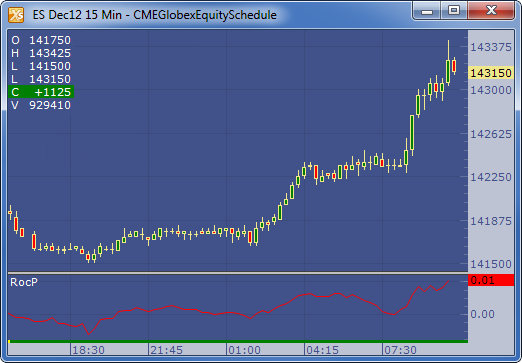← Back to X_TRADER® Help Library

### X_STUDY® Documentation

#### Rate of Change (ROCP)

##### Description

The Rate of Change Percentage (ROCP) indicator compares the current price with the previous price from a selected number of periods ago. The current price is divided by the previous price. ROCP does not express as a percentage. This indicator is also commonly known as a momentum indicator.

##### Formula

ROCP = (Current Price / Price of n bars ago)-1.0)

Where: n = Time period

##### Example